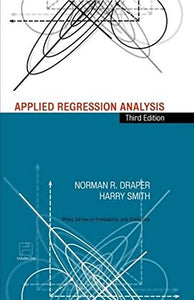Wiley-Interscience

# Applied Regression Analysis (Wiley Series In Probability And Statistics)

• Publish Date: 1998-04-23
• Binding: Hardcover
• Author: Norman R. Draper;Harry Smith

Regular price \$51.73 Sale price \$312.60

Attention: For textbook, access codes and supplements are not guaranteed with used items.

An outstanding introduction to the fundamentals of regression analysis-updated and expanded The methods of regression analysis are the most widely used statistical tools for discovering the relationships among variables. This classic text, with its emphasis on clear, thorough presentation of concepts and applications, offers a complete, easily accessible introduction to the fundamentals of regression analysis. Assuming only a basic knowledge of elementary statistics, Applied Regression Analysis, Third Edition focuses on the fitting and checking of both linear and nonlinear regression models, using small and large data sets, with pocket calculators or computers. This Third Edition features separate chapters on multicollinearity, generalized linear models, mixture ingredients, geometry of regression, robust regression, and resampling procedures. Extensive support materials include sets of carefully designed exercises with full or partial solutions and a series of true/false questions with answers. All data sets used in both the text and the exercises can be found on the companion disk at the back of the book. For analysts, researchers, and students in university, industrial, and government courses on regression, this text is an excellent introduction to the subject and an efficient means of learning how to use a valuable analytical tool. It will also prove an invaluable reference resource for applied scientists and statisticians.